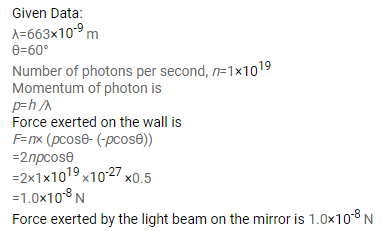# A parallel beam of monochromatic light of wavelength

Question:

A parallel beam of monochromatic light of wavelength $663 \mathrm{~nm}$ is incident on a totally reflecting plane mirror. The angle of incidence is $60^{\circ}$ and the number of photons striking the mirror per second is $1.0 \times 10^{19}$. Calculate the force exerted by the light beam on the mirror.

Solution: